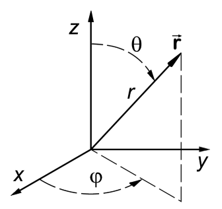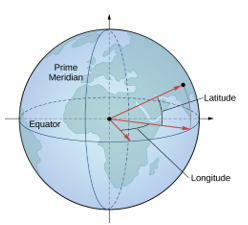# Spherical Coordinates, Convert to Cartesian & Radians to Degrees

Contents:

## Spherical Coordinates: Definition

Spherical Coordinates make up a type of Coordinate System that best suits the description of positions and overall geometry of a sphere. The system normally uses radians instead of degrees.

The Spherical Coordinate System replaces the x, y, and z Cartesian Coordinates with the following:

• X-coordinate replaced by Radial distance (r)
• Y-coordinate replaced by Polar Angle (θ)
• Z-coordinate replaced by Azimuthal Angle (φ)The referencing for each spherical coordinate (r, θ, φ) is based on the z-axis, where:

• Polar Angle is the angle made from reflecting off the z-axis.
• Azimuthal Angle is the angle made from reflecting off the x-axis and revolves on the x-y plane.

The exact placement of the spherical coordinate matches that of the Cartesian coordinate. However, both the naming of the systems and the process in getting to that coordinate differs.

## Common Convention

It is common practice to have:

• All distance length of r is kept as a positive number
• The polar angle to be in degrees or radians of limitation from 0 to 180° or π rad
• The azimuthal angle* to be in degrees or radians of limitation from 0 to 360° or 2π rad.

*The azimuthal angle is preferred to be positive to follow the positive counterclockwise direction (similar to what occurs in the unit circle).

The spherical coordinate system can be extended to dimensional spaces higher than the third dimension, referred to as the Hyperspherical Coordinate System. However, the visualization of higher-dimensional coordinate systems is limited to graphical approximations and word descriptions.

## Real-World Application

For a coordinate system that describes a point through spherical references, the spherical coordinate system is widely used to map exact points on Earth. The naming of the polar angle and azimuthal angle gets changed to latitude and longitude. The name change expresses how far above the equator and how far across from the prime meridian a point is, geographically speaking.The application use for spherical coordinates was found useful within the sound industry; specifically, for mapping frequency patterns. Simple tests for frequency levels (in Hertz) uses spherical coordinates to visualize frequency performance. The performance tests would show how balanced or distorted sound pressure distributions and sound waves are detected by human ears (Kerscher, 2010).

## Spherical Coordinates: Convert to and from Cartesian

To convert from three-dimensional Cartesian coordinates (x, y, z) to spherical coordinates (r, θ, φ), use the following formulas:To convert from spherical coordinates to Cartesian coordinates:The backward conversion has similar domain restrictions for the spherical coordinates. The restrictions have the radius r ranging from θ to infinity, the polar angle θ ranging from 0 to 90° or π radians, and the azimuth angle φ ranging from 0 to 360° or 2π radians.

Example: Convert the Cartesian coordinates (1, 2, 3) on the third-dimensional plane.

Step 1: Substitute in the given x, y, and z coordinates into the corresponding spherical coordinate formulas.Step 2: Group the spherical coordinate values into proper form.

Solution: For the Cartesian Coordinates (1, 2, 3), the Spherical-Equivalent Coordinates are (√(14), 36.7°, 63.4°).

## Uses of Spherical Coordinates

Spherical coordinates can be used to graph surfaces ranging from spheres, planes, cones, and any combination of the three.Let the Radial Distance r equation equal a constant and the Polar/Azimuthal Angles equal to their formulas. The constant Radial Distance forces the two angles to span their domain of allowable angles. The spanning of these angles forms a sphere with its radius equal to the Radial Distance value.Letting the Polar Angle θ be equal to a constant instead restricts the surface to a half-plane of, theoretically, infinite length and area. The half-plane can rotate around the z-axis and extend outwards from the origin. The spherical restriction is based on the surface to contain only one angle off from the z-axis. This means that no surface distortion or stretch is present.Lastly, setting the Azimuthal Angle φ to equal a constant restricts the surface to take the shape of a cone. The graphed shape depicts the angle distance parameter by the rotating linear line made from the origin point to the circle. The polar angle parameter dependency is visible by how narrow or wide the cone’s radius can be graphed.

Whether the cones are directed upwards or downwards depends on the direction that the azimuthal angle is set. The positive sign points the cone upward, while negative sign points the cone downward.

## Complex Surface Discussion

Three surface generalizations are made by taking one spherical coordinate parameter to equal a constant and letting the other two parameters be free to take on all values within their domain. More complex surfaces, such as a topped cone, combines a cone surface with a partially cut hemisphere.Surface visualizations like this are of interest to mathematicians to calculate the encompassed volumes and surface areas of complex surfaces. These calculations are examined by the Triple Integral concept where the integral of each parameter of the spherical coordinate system is evaluated in a specific order.

A radian is a measure of an angle. It is a SI unit, which means it was chosen as a standard unit of measurement by the General Conference on Weights and Measures.

It is defined so that on a unit circle (a circle with radius one) an angle of 1 radian will give an arc with a length of 1. Since the circumference of the entire unit circle is 2 π, 360 degrees is equal to 2 π radians. So:

• 1 radian = 180/π degrees (57.29577951), and
• 1 degree = π/180 radians.

Take any angle, and imagine the arc of a circle that it subtends (subtends is to form an angle at a particular point when straight lines from its extremities are joined at that point). Then the magnitude in radians is equal to the ratio of the arc length to the radius of the circle. For example, if the arc length was 2 and the radius of the circle was 3, the measure—in radians—would be 2/3.

Since a radian is defined as a ratio of lengths; it isn’t bound to any measurement system . It is what we call a ‘pure number’.## Notation

The most commonly used notation for a radian is simply the shortened form rad. Occasionally it is abbreviated with a simple r. Sometimes a superscript—either c or R—is used, in a way that is analogous to a degree symbol, but since it is easy to confuse a small superscript this is not a preferred method.

0.6 rad, 0.6 r, 0.6rad, 0.6c, or 0.6R are all valid and equivalent ways of writing 0.6 radians.

Since radians are ‘pure numbers’ (because they are ratios of lengths) we don’t necessarily need to designate them with ‘rad’ or any suffix at all—if you say an angle has a measure of 2, any mathematician will know you mean two radians.

To convert from radians to degrees or degrees to radians, use the basic formulas:

• Degrees to radians: Degrees x π/180.

Example question #1: What is 73° to radians?

Step 1: Insert your degrees into the formula:
Degrees to radians: Degrees x π/180 =
= 73 x π/180

That’s it!

Example question #2: What is 3 radians to degrees?

Step 1: Insert your degrees into the formula:
= 3 x 180/π
= 171.887 degrees.

That’s it!

Mathematicians like to use radians because formulas become much simpler when written in those terms. You will probably grow to like them, also, because they make Calculus much simpler. For example, if x is measured in radians, we can say that the derivative of sin x is just cos x.

## Spherical Coordinates: References

Kerscher, Michael. Compact Spherical Loudspeaker Array for Variable Sound-Radiation. 2010. Institute of Electronic Music and Acoustics, PhD dissertation. https://iem.kug.ac.at/fileadmin/media/iem/projects/2010/kerscher.pdf